Courses

# Reaction Equilibria for Simultaneous Reactions Civil Engineering (CE) Notes | EduRev

## Civil Engineering (CE) : Reaction Equilibria for Simultaneous Reactions Civil Engineering (CE) Notes | EduRev

The document Reaction Equilibria for Simultaneous Reactions Civil Engineering (CE) Notes | EduRev is a part of the Civil Engineering (CE) Course Thermodynamics.
All you need of Civil Engineering (CE) at this link: Civil Engineering (CE)

Reaction equilibria for simultaneous reactions

While we have so far presented reaction equlibria for single reactions, the more common situation that obtains in industrial practice is that of multiple, simultaneous reactions. Usually this occurs due to the presence of ‘side’ reactions that take place in addition to the main, desired reaction. This leads to the formation of unwanted side products, necessitating additional investments in the form of purification processes to achieve the required purity of the product(s). An example of such simultaneously occurring reaction is: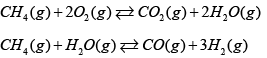Clearly the challenge in such cases is to determine the reaction conditions (of temperature, pressure and feed composition) that maximize the conversion of the reactants to the desired product(s). Essentially there are two methods to solve for the reaction equilibria in such systems.

Method 1:

Use of reaction-co-ordinates for each reaction This is an extension of the method already presented in the last section for single reactions. Consider, for generality, a system containing i chemical species, participating in j independent parallel reactions, each defined by a reaction equilibrium constant Kj and a reaction co-ordinate ξj . One can then write a set of j equations of the type 8.64 as follows: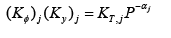...(8.68)
Where, αj and yi are given by eqns. 8.37 and 8.39 respectively (as follows):

And,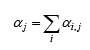...(8.37)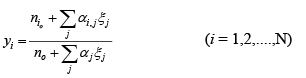...(8.39)
Therefore there are j unknown reaction co-ordinates which may be obtained by solving simultaneously j equations of the type 8.68.

Method 2:

Use of Lagrangian Undetermined Multipliers :

This method utilizes the well-known Lagrangian method of undetermined multipliers typically employed for optimizing an objective function subject to a set of constraints. As outlined in section 8.3 at the point of equilibrium in a reactive system, the total Gibbs free energy of the system is a minimum. Further, during the reaction process while the total number of moles may not be conserved, the total mass of each atomic species remains constant. Thus, in mathematical terms, the multi-reaction equilibria problem amounts to minimizing the total Gibbs free energy of the system subject to the constraint of conservation of total atomic masses in the system. The great advantage that this approach offers over the previous method is that one does not need to explicitly determine the set of independent chemical reactions that may be occurring in the system.

We formulate below the set of equations that need to be solved to obtain the composition of the system at equilibrium. Let there be N chemical (reactive) species and p (corresponding) elements in a system; further, ni = initial no of moles of species i; βik = number of atoms of kth element in the ith k chemical species; β = total number of atomic masses of  Kth   element as available in the initial feed composition.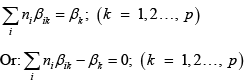...(8.69, 8.70)

Use of p number of Lagrangian multipliers (one for each element present in the system) give: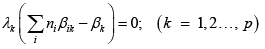...(8.71)
These equations are summed over p, giving: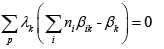...(8.72)

Let Gtbe the total Gibbs free energy of the system. Thus, incorporating p equations of the type 8.72 one can write the total Lagrangian L for the system as follows: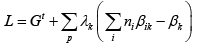...(8.73)

It may be noted that in eqn. 8.73, L always equals Gt as the second term on the RHS is identically zero. Therefore, minimum values of both Land G t occur when the partial derivatives of L with respect to all the n i and λare zero.

Thus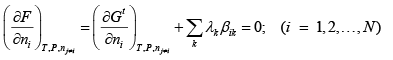...(8.74)

However, the first term on the RHS is the chemical potential of each reactive species in the system; thus eqn. 8.74 may be written as: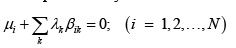...(8.75)

But by eqn. 8.44: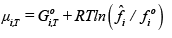...(8.44)

Once again, we consider, for illustration, the case of gaseous reactions for which the standard state pressure for each species is 1 bar, whence, fi 0 = 1bar.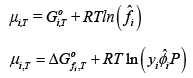...(8.76, 8.77)

In the above equation may be equated to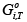may be equated to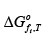the latter being the standard Gibbs free energy of formation of the ‘i’ species (at temperature T). In arriving at this relation, the standard Gibbs free energy of formation of the elements comprising the ith species are arbitrarily set to zero (for convenience of calculations). Thus combining eqns. 8.75 and 8.77 one obtains: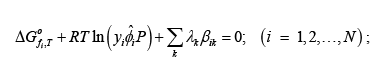...(8.78)

In eqn. 8.78, the reaction pressure P needs to be specified in bar (as fi 0 = 1bar ). Also, if the ith species is an element, the corresponding= 0

Further taking the partial derivative of the Lagrangian L (of eqn. 8.73)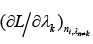with respect to each of the p undetermined multipliers, an additional set of p equations of type 8.70 obtains. Thus there are a total of ( N +p) equations which may be solved simultaneously to obtain the complete set of equilibrium mole fractions of N species.

Offer running on EduRev: Apply code STAYHOME200 to get INR 200 off on our premium plan EduRev Infinity!

## Thermodynamics

56 videos|92 docs|33 tests

,

,

,

,

,

,

,

,

,

,

,

,

,

,

,

,

,

,

,

,

,

;